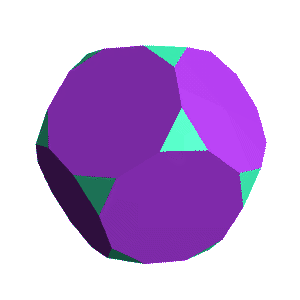Up: The Archimedean Solids

# The Dodecahedron and the Truncated DodecahedronDodecahedron 12 pentagonal faces20 verticesTruncated Dodecahedron 20 10-sided faces20 triangular faces

### What's happening?

To cut off the corners of the dodecahedron, we move in the same distance from each corner along the edges. The distance we move is less than half of the side length, because it is impossible to move in more. (If we move in exactly half way, the result is the icosidodecahedron)

This process shortens the side lengths of the edges in the original dodecahedron by the same amount. Notice that it also doubles the number of edges -- changing the purple pentagonal faces of the dodedahedron (left) into purple 10-sided faces in the truncated dodecahedron (right).

In the dodecahedron, three faces meet at every vertex. Cutting off the original vertices, then, leaves a triangular shape behind, which is filled in by the green triangles.

Next: Cuboctahedron
Go back to The Archimedean Solids